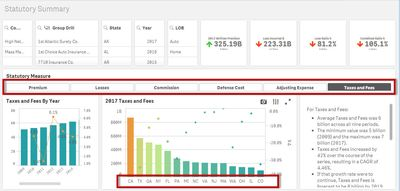# Qlik Sense App Development

cancel
Showing results for
Did you mean:
HighlightedCreator II

## Aggr Dimension with Pick

Hello I have a bar chart with an aggr dimension to capture the top 15 states by the sum of a value.  I would like the top 15 to flex based on the value of a selected variable.  In my sheet i have a variable that allows the user to select a measure and the chart flexes to that measure.  I want the aggr to flex to that measure as well.  It is as if i need to embed the variable within the aggr but I can't get it to work.  Below my screen print I have pasted the set analysis that drives the metrics/visualizations.  Any help would be much appreciated.Here are each of the metrics behind the button/variable.

pick(vDashboard,

sum({<DATA_TYPE={STAT}, [Year]={\$(=max([Year]))}>}INC_LOSSES*1000), //Incurred Losses

sum({<DATA_TYPE={STAT}, [Year]={\$(=max([Year]))}>}COMM_BROK_EXP*1000), //Commission

sum({<DATA_TYPE={STAT}, [Year]={\$(=max([Year]))}>}DCC_EXP_INC*1000), //Defense Cost

sum({<DATA_TYPE={STAT}, [Year]={\$(=max([Year]))}>}TAX_LIC_FEES*1000) //Taxes and Fees

)

Here's my current aggr function for state:

I tried this but it didn't work:

=if(aggr(rank((pick(vDashboard,

sum({<DATA_TYPE={STAT}, [Year]={\$(=Max([Year]))}>}INC_LOSSES*1000), //Incurred Losses

sum({<DATA_TYPE={STAT}, [Year]={\$(=Max([Year]))}>}COMM_BROK_EXP*1000), //Commission

sum({<DATA_TYPE={STAT}, [Year]={\$(=Max([Year]))}>}DCC_EXP_INC*1000), //Defense Cost

sum({<DATA_TYPE={STAT}, [Year]={\$(=Max([Year]))}>}TAX_LIC_FEES*1000) //Taxes and Fees

))
,STATE)<=15,STATE)

Labels (8)

• ### variable

1 Solution

Accepted Solutions
HighlightedCreator II

## Re: Aggr Dimension with Pick

Turns out I was on the right track...  A single open parenthesis tripped the whole thing up.

=if(aggr(rank(pick(vDashboard,

sum({<DATA_TYPE={STAT}, [Year]={\$(=Max([Year]))}>}INC_LOSSES*1000)/12, //Incurred Losses

sum({<DATA_TYPE={STAT}, [Year]={\$(=Max([Year]))}>}COMM_BROK_EXP*1000)/12, //Commission

sum({<DATA_TYPE={STAT}, [Year]={\$(=Max([Year]))}>}DCC_EXP_INC*1000)/12, //Defense Cost

sum({<DATA_TYPE={STAT}, [Year]={\$(=Max([Year]))}>}TAX_LIC_FEES*1000)/12 //Taxes and Fees

)),STATE)<=15,STATE)

HighlightedCreator II

## Re: Aggr Dimension with Pick

Turns out I was on the right track...  A single open parenthesis tripped the whole thing up.

=if(aggr(rank(pick(vDashboard,